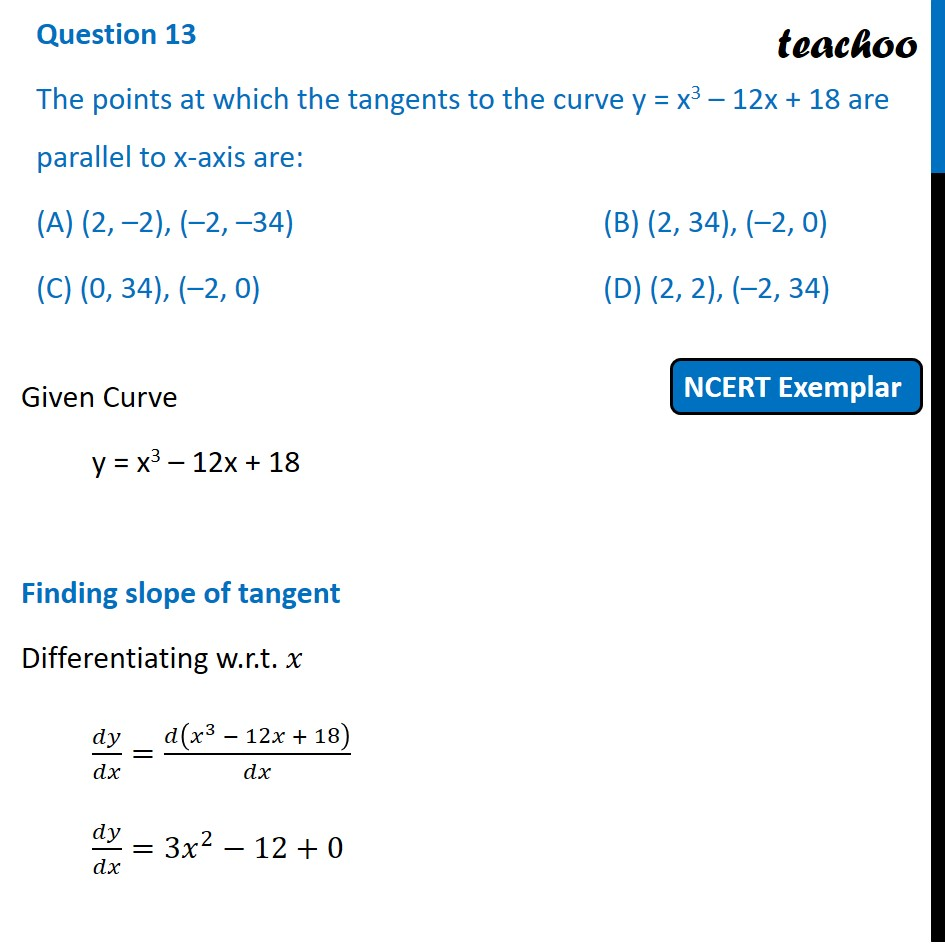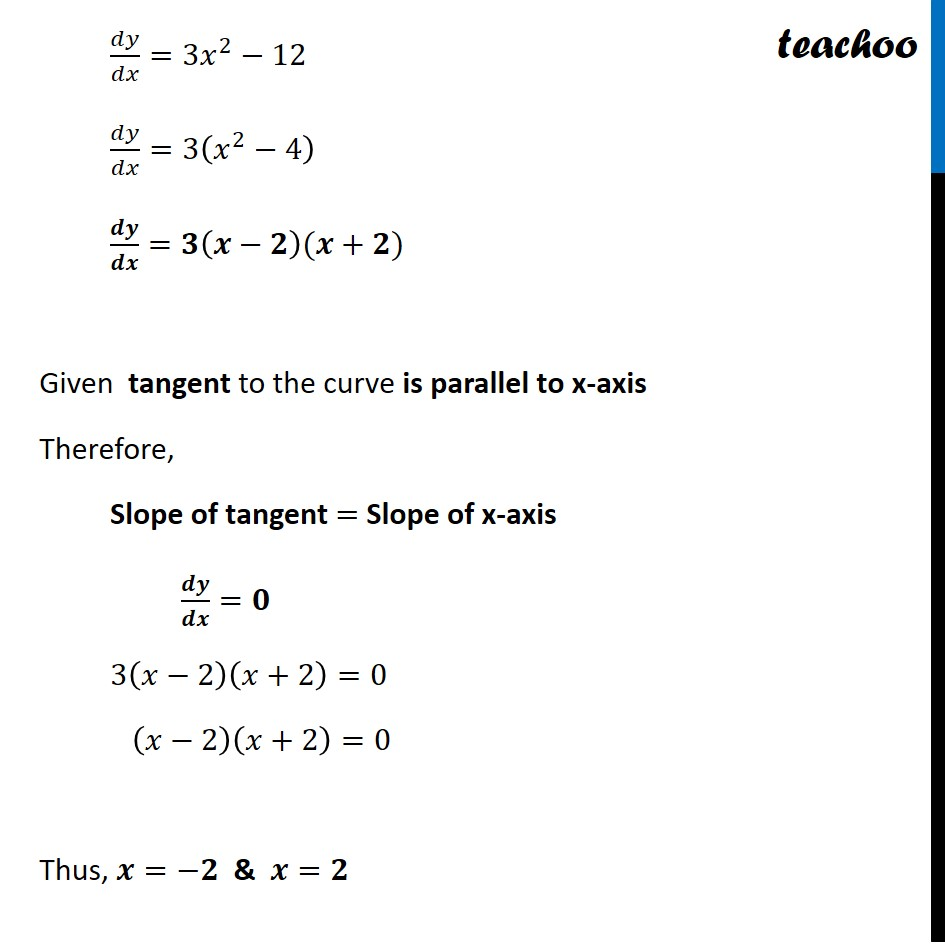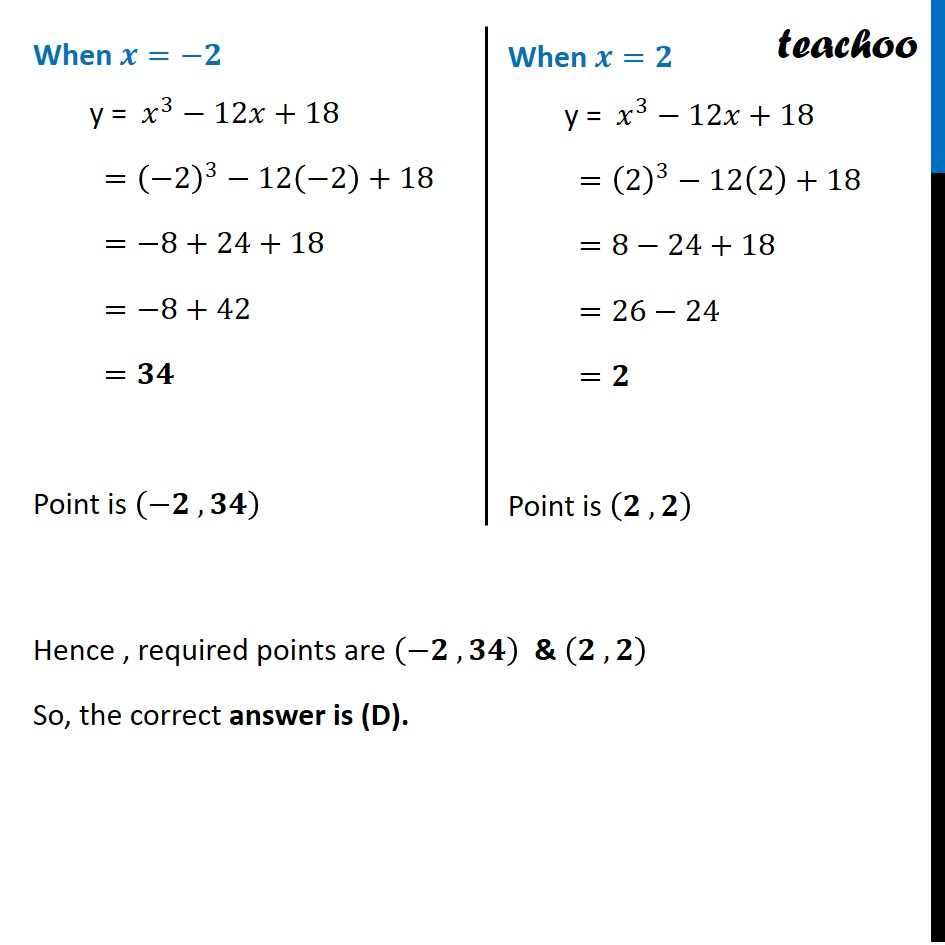NCERT Exemplar - MCQs

Chapter 6 Class 12 Application of Derivatives
Serial order wise

## (D) (2, 2), (–2, 34)

This question is similar to Ex 6.3, 7 - Chapter 6 Class 12 - Application of DerivativesLearn in your speed, with individual attention - Teachoo Maths 1-on-1 Class

### Transcript

Question 11 The points at which the tangents to the curve y = x3 – 12x + 18 are parallel to x-axis are: (A) (2, –2), (–2, –34) (B) (2, 34), (–2, 0) (C) (0, 34), (–2, 0) (D) (2, 2), (–2, 34) Given Curve "y = x3 – 12x + 18" Finding slope of tangent Differentiating w.r.t. 𝑥 𝑑𝑦/𝑑𝑥=𝑑(𝑥^3 − 12𝑥 + 18)/𝑑𝑥 𝑑𝑦/𝑑𝑥=3𝑥^2−12+0 𝑑𝑦/𝑑𝑥=3𝑥^2−12 𝑑𝑦/𝑑𝑥=3(𝑥^2−4) 𝒅𝒚/𝒅𝒙=𝟑(𝒙−𝟐)(𝒙+𝟐) Given tangent to the curve is parallel to x-axis Therefore, Slope of tangent = Slope of x-axis 𝒅𝒚/𝒅𝒙=𝟎 3(𝑥−2)(𝑥+2)=0 (𝑥−2)(𝑥+2)=0 Thus, 𝒙=−𝟐 & 𝒙=𝟐 When 𝒙=−𝟐 "y = " 𝑥^3−12𝑥+18 =(−2)^3−12(−2)+18 =−8+24+18 =−8+42 =𝟑𝟒 Point is (−𝟐 , 𝟑𝟒) When 𝒙=𝟐 "y = " 𝑥^3−12𝑥+18 =(2)^3−12(2)+18 =8−24+18 =26−24 =𝟐 Point is (𝟐 , 𝟐) Hence , required points are (−𝟐 , 𝟑𝟒) & (𝟐 , 𝟐) So, the correct answer is (D).